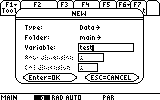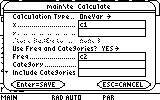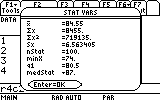# Knowledge Base

## Solution 12116: Calculating Weighted Mean Using the TI-89 Family, TI-92 Plus and Voyage™ 200 Graphing Calculators.

### How do I calculate the weighted mean using the TI-89 family, TI-92 Plus or Voyage 200 graphing calculator?

Weighted mean is the mean of a data set whose entries have varying weights. This is a one variable statistical calculation involving 2 lists. Refer to the example below for calculating weighted mean using the TI-89 family, TI-92 Plus and Voyage 200 graphing calculators.

Example : Suppose a student's grade in a class is determined as follows: 65% is from the test mean, 25% is from the final exam, and 10% is from homework. If the student's test mean is 87 and the final exam grade is 74 and the homework grade is 95, what is the weighted mean of the student´s scores?

X values: {87, 74, 95}

Y values: {65, 25, 10}

Refer to the steps provided below to calculate the student´s weighted mean in this class:

1) Press [APPS]  . (If the Apps Desktop is on, press [APPS], select "Data/Matrix Editor").

2) Scroll down to "3:New" and press [ENTER].

3) In the box beside "Variable:", type a variable name using the alpha characters (e.g. test).4) Press [ENTER] [ENTER].

5) Enter the X values under c1 and the Y values under c2.

6) Press [F5], then the right arrow, then  for "One-Var".

7) Press the down arrow, and then press [C] [alpha]  (indicates the Y values are in c1).

8) Pressing the down arrow, highlight "Use Freq and Categories", then press the right arrow and select "2:YES".

9) Press the down arrow, then press [C] [alpha]  (indicates the frequencies are in c2).10) Press [ENTER] [ENTER] to display the results.The student´s grade in this class or the weighted mean is 84.55.

Please see the TI-89 family, TI-92 family and Voyage 200 guidebooks for additional information.